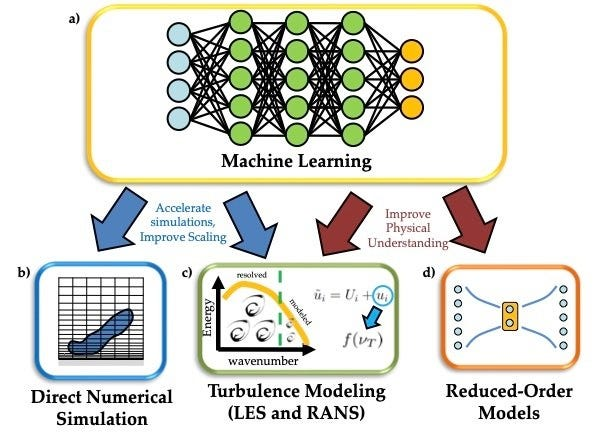# Decoding the Outcome: An In-depth Guide to Understanding and Leveraging Machine Learning Results### Introduction

Machine learning has emerged as a powerful tool in today’s data-driven world. As more organizations harness the power of machine learning to gain insights and make predictions from their data, it’s crucial to understand not just how to create machine learning models, but how to interpret and effectively use their results. This comprehensive guide is designed to help data practitioners understand and leverage the results generated by machine learning models to make informed decisions.

### Understanding Machine Learning Results

At a fundamental level, machine learning is about using algorithms to learn from data and make predictions or decisions. The ‘learning’ occurs when a machine learning model is trained on a dataset and then produces an output based on that learning when it encounters new data. The output of a machine learning model is known as the ‘result’.

Machine learning results can take several forms, depending on the type of task the model is designed to perform:

Classification: If the machine learning model is a classifier, it classifies input data into predefined categories or classes. The result is a label representing the class to which the input data belongs.

Regression: If the machine learning model is designed for regression, it predicts a continuous outcome. The result is a numerical value representing the prediction.

Clustering: If the model is a clustering algorithm, it groups similar data together. The result is a set of clusters, each containing data points that are similar to each other.

Association Rule Learning: In this case, the model finds associations or correlation relationships among a set of items. The result is a set of rules highlighting these associations.

### How to Interpret Machine Learning Results

Interpreting the results of a machine learning model is a crucial step that requires understanding the problem domain, the data, and the type of machine learning model used. Here’s how to interpret results based on the type of task:

Classification: The predicted class label needs to be evaluated in the context of the problem domain. Metrics like accuracy, precision, recall, F1-score, and area under the ROC curve are commonly used to assess the performance of a classifier.

Regression: The predicted numerical value should be interpreted in the context of the problem. Metrics like mean absolute error, mean squared error, root mean squared error, and R-squared are often used to evaluate regression models.

Clustering: The clusters generated by the algorithm should be analyzed to identify common characteristics within each cluster and differences between clusters. Silhouette score, Davies-Bouldin index, and Dunn index are common metrics to evaluate clustering algorithms.

Association Rule Learning: The generated rules need to be analyzed based on their support, confidence, and lift to determine their significance and usefulness.

### Leveraging Machine Learning Results

Machine learning results can be leveraged in various ways depending on the problem at hand:

Decision Making: Machine learning results can inform decision-making processes. For instance, a classifier predicting customer churn can help devise retention strategies.

Forecasting: Regression models can forecast future values, aiding in planning and resource allocation.

Insights and Understanding: Clustering and association rule learning can provide insights into patterns and relationships within the data, contributing to a better understanding of the problem domain.

Automation: Machine learning models can automate tasks that would be too time-consuming or difficult for humans, increasing efficiency.

### Conclusion

Understanding and leveraging machine learning results is a critical aspect of applying machine learning effectively. By understanding what the results represent, how to interpret them, and how they can be used, you can turn machine learning outputs into actionable insights, informed decisions, and effective solutions. The goal is to utilize these

models to create real-world impact, and the first step towards that is comprehending the results that these models produce. This comprehensive guide provides you with the necessary understanding to interpret and utilize the results of your machine learning models effectively.

# Personal Career & Learning Guide for Data Analyst, Data Engineer and Data Scientist

## Applied Machine Learning & Data Science Projects and Coding Recipes for Beginners

A list of FREE programming examples together with eTutorials & eBooks @ SETScholars

# Projects and Coding Recipes, eTutorials and eBooks: The best All-in-One resources for Data Analyst, Data Scientist, Machine Learning Engineer and Software Developer

Topics included:Classification, Clustering, Regression, Forecasting, Algorithms, Data Structures, Data Analytics & Data Science, Deep Learning, Machine Learning, Programming Languages and Software Tools & Packages.
(Discount is valid for limited time only)## Find more … …

Leveraging AI-Powered Tools in Microsoft 365 to Optimize Workday Productivity and Efficiency

Year Five Math Worksheet for Kids – Interpreting Fractions as Division

Excel to Python Example – How to count rows if meet multiple criteria or business rules